Theory and Modern Applications

# A new transform method in nabla discrete fractional calculus

## Abstract

Starting from the definition of the Sumudu transform on a general nabla time scale, we define the generalized nabla discrete Sumudu transform. We obtain the nabla discrete Sumudu transform of Taylor monomials, fractional sums, and differences. We apply this transform to solve some fractional difference equations with initial value problems.

MSC:44A15, 44A55.

## 1 Introduction

The fractional calculus deals with integrals and derivatives of arbitrary orders. Many scientists have paid a lot of attention to this calculus because of its interesting applications in various fields of science, such as viscoelasticity, diffusion, neurology, control theory, and statistics; see .

The analogous theory for discrete fractional calculus was initiated by Miller and Ross , where basic approaches, definitions, and properties of the theory of fractional sums and differences were reported. Recently, a series of papers continuing this research has appeared. We refer the reader to the papers  and the references cited therein.

In the early 1990s, Watugala [17, 18] introduced the Sumudu transform and applied it to solve ordinary differential equations. The fundamental properties of this transform, which is thought to be an alternative to the Laplace transform, were then established in many articles .

The Sumudu transform is defined over the set of functions

by

$F\left(u\right):=\mathbb{S}\left\{f\right\}\left(u\right):=\frac{1}{u}{\int }_{0}^{\mathrm{\infty }}f\left(t\right){e}^{-\frac{t}{u}}\phantom{\rule{0.2em}{0ex}}dt,\phantom{\rule{1em}{0ex}}u\in \left(-{\tau }_{1},{\tau }_{2}\right).$

Without doubt, the Sumudu transform of a function has a deep connection to its Laplace transform. However, the Sumudu transform is a bit superior as it may be used to solve problems without resorting to a new frequency domain because it preserves scales and unit properties. By these properties, the Sumudu transform may be used to solve intricate problems in engineering and applied sciences that can hardly be solved when the Laplace transform is used. Moreover, some properties of the Sumudu transform makes it more advantageous than the Laplace transform. Some of these properties are as follows.

1. (1)

$\mathbb{S}\left\{{t}^{n}\right\}\left(u\right)=n!{u}^{n}$.

2. (2)

${lim}_{u\to -{\tau }_{1}}F\left(u\right)={lim}_{t\to -\mathrm{\infty }}f\left(t\right)$.

3. (3)

${lim}_{u\to {\tau }_{2}}F\left(u\right)={lim}_{t\to \mathrm{\infty }}f\left(t\right)$.

4. (4)

${lim}_{t\to {0}^{\mp }}f\left(t\right)={lim}_{u\to {0}^{\mp }}F\left(u\right)$.

5. (5)

For any real or complex number c, $\mathbb{S}\left\{f\left(ct\right)\right\}\left(u\right)=F\left(cu\right)$.

Particularly, since constants are fixed by the Sumudu transform, choosing $c=0$, it gives $F\left(0\right)=f\left(0\right)$.

In dealing with physical applications, this aspect becomes a major advantage, especially in instances where keeping track of units and dimensional factor groups of constants is relevant. This means that in problem solving, u and $F\left(u\right)$ can be treated as replicas of t and $f\left(t\right)$, respectively .

Recently, an application of the Sumudu and double Sumudu transforms to Caputo fractional differential equations is given in . In , the authors applied the Sumudu transform to fractional differential equations.

In , the authors obtained the discrete Sumudu transform of Taylor monomials, fractional sums, and fractional differences and applied this transform to solve a fractional difference initial value problem.

Starting with a general definition of the Laplace transform on an arbitrary time scale, the concepts of the h-Laplace and consequently the discrete Laplace transform were specified in . The theory of time scales was initiated by Stefan Hilger . This theory is a tool that unifies the theories of continuous and discrete time systems. It is a subject of recent studies in many different fields in which a dynamic process can be described with discrete or continuous models.

In this paper, starting from the definition of the Sumudu transform on a general nabla time scale, we define the nabla discrete Sumudu transform and present some of its basic properties.

The paper is organized as follows. In Sections 2 and 3, we introduce some basic concepts concerning the calculus of time scales and discrete fractional calculus, respectively. In Section 4, we define the nabla discrete Sumudu transform and present some of its basic properties. Section 5 is devoted to some applications.

## 2 Preliminaries on time scales

A time scale $\mathbb{T}$ is an arbitrary nonempty closed subset of the real numbers . The most well-known examples are $\mathbb{T}=\mathbb{R}$, $\mathbb{T}=\mathbb{Z}$, and $\mathbb{T}=\overline{{q}^{\mathbb{Z}}}:=\left\{{q}^{n}:n\in \mathbb{Z}\right\}\cup \left\{0\right\}$, where $q>1$. The forward and backward jump operators are defined by

$\sigma \left(t\right):=inf\left\{s\in \mathbb{T}:s>t\right\}\phantom{\rule{1em}{0ex}}\text{and}\phantom{\rule{1em}{0ex}}\rho \left(t\right):=sup\left\{s\in \mathbb{T}:s

respectively, where $inf\mathrm{\varnothing }:=sup\mathbb{T}$ and $sup\mathrm{\varnothing }:=inf\mathbb{T}$. A point $t\in \mathbb{T}$ is said to be left-dense if $t>inf\mathbb{T}$ and $\rho \left(t\right)=t$, right-dense if $t and $\sigma \left(t\right)=t$, left-scattered if $\rho \left(t\right), and right-scattered if $\sigma \left(t\right)>t$. The backwards graininess function $\nu :\mathbb{T}\to \left[0,\mathrm{\infty }\right)$ is defined by $\nu \left(t\right):=t-\rho \left(t\right)$. For details, see the monographs [30, 31].

The following two concepts are introduced in order to describe the classes of functions that are integrable.

Definition 2.1 

A function $f:\mathbb{T}\to \mathbb{R}$ is called regulated if its right-sided limits exist at all right-dense points in $\mathbb{T}$ and its left-sided limits exist at all left-dense points in $\mathbb{T}$.

Definition 2.2 

A function $f:\mathbb{T}\to \mathbb{R}$ is called ld-continuous if it is continuous at left-dense points in $\mathbb{T}$ and its right-sided limits exist at right-dense points in $\mathbb{T}$.

The set ${\mathbb{T}}_{\kappa }$ is derived from the time scale $\mathbb{T}$ as follows: If $\mathbb{T}$ has a right-scattered minimum m, then ${\mathbb{T}}_{\kappa }:=\mathbb{T}-\left\{m\right\}$. Otherwise, ${\mathbb{T}}_{\kappa }:=\mathbb{T}$.

Definition 2.3 

A function $f:\mathbb{T}\to \mathbb{R}$ is said to be nabla differentiable at a point $t\in {\mathbb{T}}_{\kappa }$ if there exists a number ${f}^{\mathrm{\nabla }}\left(t\right)$ with the property that given any $\epsilon >0$, there exists a neighborhood U of t such that

We shall also need the following definition in order to define the nabla exponential function on an arbitrary time scale.

Definition 2.4 

A function $p:\mathbb{T}\to \mathbb{R}$ is called ν-regressive provided $1-\nu \left(t\right)p\left(t\right)\ne 0$ for all $t\in {\mathbb{T}}_{\kappa }$.

The set ${\mathcal{R}}_{\nu }$ of all ν-regressive and ld-continuous functions forms an Abelian group under the ‘circle plus’ addition defined by

The additive inverse p of $p\in {\mathcal{R}}_{\nu }$ is defined by

Theorem 2.5 

Let $p\in \mathcal{R}$ and ${t}_{0}\in \mathbb{T}$ be a fixed point. Then the nabla exponential function ${e}_{p}\left(\cdot ,{t}_{0}\right)$ is the unique solution of the initial value problem

${y}^{\mathrm{\nabla }}=p\left(t\right)y,\phantom{\rule{2em}{0ex}}y\left({t}_{0}\right)=1.$

We next define the nabla Taylor monomials and later generalize them for noninteger orders.

Definition 2.6 

The Taylor monomials are the functions ${h}_{n}:\mathbb{T}×\mathbb{T}\to \mathbb{R}$, $n\in {\mathbb{N}}_{0}$, and are defined recursively as follows:

and for $n\in {\mathbb{N}}_{0}$,

Throughout, we shall consider the discrete time scale

In this case, when $p\left(t\right)\equiv p$, where $p\in \mathcal{R}:=\mathbb{C}\setminus \left\{1\right\}$ and ${t}_{0}=a$, it is easy to see that

${e}_{p}\left(t,a\right)={\left(1-p\right)}^{a-t},$

and for all nonnegative integers n,

${h}_{n}\left(t,a\right)=\frac{{\left(t-a\right)}^{\overline{n}}}{n!}.$

## 3 An introduction to nabla discrete fractional calculus

For any function $f:{\mathbb{N}}_{a}\to \mathbb{R}$, we define the backwards difference, or nabla operator, , by $\left(\mathrm{\nabla }f\right)\left(t\right):=f\left(t\right)-f\left(t-1\right)$ for $t\in {\mathbb{N}}_{a+1}$. In this paper, we use the convention that $\mathrm{\nabla }f\left(t\right):=\left(\mathrm{\nabla }f\right)\left(t\right)$. We then define higher order differences recursively by ${\mathrm{\nabla }}^{n}f\left(t\right):=\mathrm{\nabla }\left({\mathrm{\nabla }}^{n-1}f\left(t\right)\right)$ for $t\in {\mathbb{N}}_{a+n}$, $n\in \mathbb{N}$. In addition, we take ${\mathrm{\nabla }}^{0}$ as the identity operator.

Definition 3.1 

Let $f:{\mathbb{N}}_{a}\to \mathbb{R}$ and $\alpha >0$ be given. Then the α th-order nabla fractional sum of f is given by

Also, we define the trivial sum by ${\mathrm{\nabla }}_{a}^{-0}f\left(t\right):=f\left(t\right)$ for $t\in {\mathbb{N}}_{a}$.

The term ${\left(t-\rho \left(s\right)\right)}^{\overline{\alpha -1}}$ in the above definition is the so-called generalized rising function defined by

${t}^{\overline{\alpha }}:=\frac{\mathrm{\Gamma }\left(t+\alpha \right)}{\mathrm{\Gamma }\left(t\right)}$

for any $t,\alpha \in \mathbb{R}$ for which the right-hand side is well defined. As usual, we use the convention that if $t\in \left\{0,-1,-2,\dots \right\}$ and $t+\alpha \notin \left\{0,-1,-2,\dots \right\}$, then ${t}^{\overline{\alpha }}:=0$.

Definition 3.2 

Let $f:{\mathbb{N}}_{a}\to \mathbb{R}$ and $\alpha >0$ be given, and let $N\in \mathbb{N}$ be chosen such that $N-1<\alpha \le N$. Then the α th-order Riemann-Liouville nabla fractional difference of f is given by

For $\alpha =0$, we set ${\mathrm{\nabla }}_{a}^{0}f\left(t\right):=f\left(t\right)$ for $t\in {\mathbb{N}}_{a}$.

As stated in the following two theorems, the composition of fractional operators behaves well if the inner operator is a fractional difference.

Theorem 3.3 

Let $\alpha ,\mu >0$. Then

${\mathrm{\nabla }}_{a}^{-\alpha }{\mathrm{\nabla }}_{a}^{-\mu }f\left(t\right)={\mathrm{\nabla }}_{a}^{-\alpha -\mu }f\left(t\right).$
(3.1)

Theorem 3.4 

For $\alpha ,\mu >0$,

${\mathrm{\nabla }}_{a}^{\alpha }{\mathrm{\nabla }}_{a}^{-\mu }f\left(t\right)={\mathrm{\nabla }}_{a}^{\alpha -\mu }f\left(t\right).$
(3.2)

Theorem 3.5 Let $\alpha >0$ and $k\in {\mathbb{N}}_{0}$ be given. Then

${\mathrm{\nabla }}_{a+k}^{-\alpha }{\mathrm{\nabla }}^{k}f\left(t\right)={\mathrm{\nabla }}_{a}^{k-\alpha }f\left(t\right)-\sum _{j=0}^{k-1}\frac{{\mathrm{\nabla }}^{j}f\left(a+j+1\right)}{\mathrm{\Gamma }\left(\alpha -k+j+1\right)}{\left(t-a-j\right)}^{\overline{\alpha -k+j}}\phantom{\rule{1em}{0ex}}\mathit{\text{for}}t\in {\mathbb{N}}_{a+k}.$
(3.3)

Proof Let $k\in {\mathbb{N}}_{0}$ be given and suppose $\alpha \notin \left\{1,2,\dots ,k-1\right\}$. We first state the following identity which follows from the product rule for the nabla operator:

${\mathrm{\nabla }}_{s}\left[{\left(t-\rho \left(s\right)\right)}^{\overline{\alpha -1}}f\left(s\right)\right]={\left(t-\rho \left(s\right)\right)}^{\overline{\alpha -1}}{\mathrm{\nabla }}_{s}f\left(s\right)-\left(\alpha -1\right){\left(t+1-\rho \left(s\right)\right)}^{\overline{\alpha -2}}f\left(\rho \left(s\right)\right).$

Then we have

$\begin{array}{rcl}{\mathrm{\nabla }}_{a+k}^{-\alpha }{\mathrm{\nabla }}^{k}f\left(t\right)& =& \frac{1}{\mathrm{\Gamma }\left(\alpha \right)}\sum _{s=a+k+1}^{t}{\left(t-\rho \left(s\right)\right)}^{\overline{\alpha -1}}{\mathrm{\nabla }}^{k}f\left(s\right)\\ =& \frac{{\left(t-\rho \left(s\right)\right)}^{\overline{\alpha -1}}}{\mathrm{\Gamma }\left(\alpha \right)}{\mathrm{\nabla }}^{k-1}f\left(s\right){|}_{a+k}^{t}+\frac{\left(\alpha -1\right)}{\mathrm{\Gamma }\left(\alpha \right)}\sum _{s=a+k+1}^{t}{\left(t+1-\rho \left(s\right)\right)}^{\overline{\alpha -2}}{\mathrm{\nabla }}^{k-1}f\left(\rho \left(s\right)\right)\\ =& {\mathrm{\nabla }}^{k-1}f\left(t\right)-\frac{{\left(t-a-k+1\right)}^{\overline{\alpha -1}}}{\mathrm{\Gamma }\left(\alpha \right)}{\mathrm{\nabla }}^{k-1}f\left(a+k\right)\\ +\frac{1}{\mathrm{\Gamma }\left(\alpha -1\right)}\sum _{s=a+k}^{t-1}{\left(t-\rho \left(s\right)\right)}^{\overline{\alpha -2}}{\mathrm{\nabla }}^{k-1}f\left(s\right)\\ =& -\frac{{\left(t-a-k+1\right)}^{\overline{\alpha -1}}}{\mathrm{\Gamma }\left(\alpha \right)}{\mathrm{\nabla }}^{k-1}f\left(a+k\right)+\frac{1}{\mathrm{\Gamma }\left(\alpha -1\right)}\sum _{s=a+k}^{t}{\left(t-\rho \left(s\right)\right)}^{\overline{\alpha -2}}{\mathrm{\nabla }}^{k-1}f\left(s\right)\\ =& {\mathrm{\nabla }}_{a+k-1}^{-\left(\alpha -1\right)}{\mathrm{\nabla }}^{k-1}f\left(t\right)-\frac{{\left(t-a-k+1\right)}^{\overline{\alpha -1}}}{\mathrm{\Gamma }\left(\alpha \right)}{\mathrm{\nabla }}^{k-1}f\left(a+k\right)\\ =& {\mathrm{\nabla }}_{a+k-2}^{-\left(\alpha -2\right)}{\mathrm{\nabla }}^{k-2}f\left(t\right)-\frac{{\left(t-a-k+2\right)}^{\overline{\alpha -2}}}{\mathrm{\Gamma }\left(\alpha -1\right)}{\mathrm{\nabla }}^{k-2}f\left(a+k-1\right)\\ -\frac{{\left(t-a-k+1\right)}^{\overline{\alpha -1}}}{\mathrm{\Gamma }\left(\alpha \right)}{\mathrm{\nabla }}^{k-1}f\left(a+k\right).\end{array}$

Continuing in this manner and summing by parts $k-2$ more times yields

${\mathrm{\nabla }}_{a+k}^{-\alpha }{\mathrm{\nabla }}^{k}f\left(t\right)={\mathrm{\nabla }}_{a}^{k-\alpha }f\left(t\right)-\sum _{j=0}^{k-1}\frac{{\mathrm{\nabla }}^{j}f\left(a+j+1\right)}{\mathrm{\Gamma }\left(\alpha -k+j+1\right)}{\left(t-a-j\right)}^{\overline{\alpha -k+j}}.$

Now assume that $\alpha \in \left\{1,2,\dots ,k-1\right\}$. From (3.1), (3.2), and the previous case, we have

$\begin{array}{rcl}{\mathrm{\nabla }}_{a+k}^{-\alpha }{\mathrm{\nabla }}^{k}f\left(t\right)& =& {\mathrm{\nabla }}^{k-\alpha }{\mathrm{\nabla }}^{-\left(k-\alpha \right)}{\mathrm{\nabla }}_{a+k}^{-\alpha }{\mathrm{\nabla }}^{k}f\left(t\right)\\ =& {\mathrm{\nabla }}^{k-\alpha }{\mathrm{\nabla }}^{-k}{\mathrm{\nabla }}^{k}f\left(t\right)\\ =& {\mathrm{\nabla }}^{k-\alpha }\left[f\left(t\right)-\sum _{j=0}^{k-1}\frac{{\mathrm{\nabla }}^{j}f\left(a+j+1\right)}{\mathrm{\Gamma }\left(j+1\right)}{\left(t-a-j\right)}^{\overline{j}}\right]\\ =& {\mathrm{\nabla }}^{k-\alpha }f\left(t\right)-\sum _{j=0}^{k-1}\frac{{\mathrm{\nabla }}^{j}f\left(a+j+1\right)}{\mathrm{\Gamma }\left(j+1\right)}{\mathrm{\nabla }}^{k-\alpha }{\left(t-a-j\right)}^{\overline{j}}\\ =& {\mathrm{\nabla }}^{k-\alpha }f\left(t\right)-\sum _{j=0}^{k-1}\frac{{\mathrm{\nabla }}^{j}f\left(a+j+1\right)}{\mathrm{\Gamma }\left(j+1\right)}\frac{\mathrm{\Gamma }\left(j+1\right)}{\mathrm{\Gamma }\left(j-\left(k-\alpha -1\right)\right)}{\left(t-a-j\right)}^{\overline{j-\left(k-\alpha \right)}}\\ =& {\mathrm{\nabla }}^{k-\alpha }f\left(t\right)-\sum _{j=0}^{k-1}\frac{{\mathrm{\nabla }}^{j}f\left(a+j+1\right)}{\mathrm{\Gamma }\left(\alpha -k+j+1\right)}{\left(t-a-j\right)}^{\overline{\alpha -k+j}}\end{array}$

with allowance for the convention $\frac{1}{\mathrm{\Gamma }\left(-k\right)}=0$ for $k\in {\mathbb{N}}_{0}$. This completes the proof. □

A disadvantage of the Riemann-Liouville fractional difference operator is that when applied to a constant c, it does not yield 0. For example, for $0<\alpha <1$, we have

${\mathrm{\nabla }}_{a}^{\alpha }c=\frac{c{\left(t-a\right)}^{\overline{1-\alpha }}}{\mathrm{\Gamma }\left(1-\alpha \right)}.$

In order to overcome this and to make the fractional differences behave like the usual differences, we define the Caputo nabla fractional difference operator, different from the definitions given in  and , as follows.

Definition 3.6 Let $f:{\mathbb{N}}_{a}\to \mathbb{R}$ and $\alpha >0$ be given, and let $N\in \mathbb{N}$ be chosen such that $N-1<\alpha \le N$. Then the α th-order Caputo nabla fractional difference of f is given by

For $\alpha =0$, we set ${}^{C}\mathrm{\nabla }_{a}^{0}f\left(t\right):=f\left(t\right)$ for $t\in {\mathbb{N}}_{a}$.

Now it follows from the definition of the Caputo nabla fractional difference operator that for $\alpha >0$, we have

${}^{C}\mathrm{\nabla }_{a}^{\alpha }c=0.$

Corollary 3.7 Let $f:{\mathbb{N}}_{a}\to \mathbb{R}$ and $\alpha >0$ be given, and let $N\in \mathbb{N}$ be chosen such that $N-1<\alpha \le N$. Then

${}^{C}\mathrm{\nabla }_{a}^{\alpha }f\left(t\right)={\mathrm{\nabla }}_{a}^{\alpha }f\left(t\right)-\sum _{j=0}^{N-1}\frac{{\mathrm{\nabla }}^{j}f\left(a+j+1\right)}{\mathrm{\Gamma }\left(-\alpha +j+1\right)}{\left(t-a-j\right)}^{\overline{-\alpha +j}}.$

Proof

By using Theorem 3.5, we see that

$\begin{array}{rcl}{}^{C}\mathrm{\nabla }_{a}^{\alpha }f\left(t\right)& =& {\mathrm{\nabla }}_{a+N}^{-\left(N-\alpha \right)}{\mathrm{\nabla }}^{N}f\left(t\right)\\ =& {\mathrm{\nabla }}_{a}^{\alpha }f\left(t\right)-\sum _{j=0}^{N-1}\frac{{\mathrm{\nabla }}^{j}f\left(a+j+1\right)}{\mathrm{\Gamma }\left(-\alpha +j+1\right)}{\left(t-a-j\right)}^{\overline{-\alpha +j}}.\end{array}$

□

Corollary 3.8 Let $f:{\mathbb{N}}_{a}\to \mathbb{R}$ and $\alpha >0$ be given, and let $N\in \mathbb{N}$ be chosen such that $N-1<\alpha \le N$. Then

${{\mathrm{\nabla }}_{a+N}^{-\alpha }}^{C}{\mathrm{\nabla }}_{a}^{\alpha }f\left(t\right)=f\left(t\right)-\sum _{j=0}^{N-1}\frac{{\mathrm{\nabla }}^{j}f\left(a+j+1\right)}{j!}{\left(t-a-j\right)}^{\overline{j}}.$

Proof

$\begin{array}{rcl}{{\mathrm{\nabla }}_{a+N}^{-\alpha }}^{C}{\mathrm{\nabla }}_{a}^{\alpha }f\left(t\right)& =& {\mathrm{\nabla }}_{a+N}^{-\alpha }{\mathrm{\nabla }}_{a+N}^{-\left(N-\alpha \right)}{\mathrm{\nabla }}^{N}f\left(t\right)={\mathrm{\nabla }}^{-N}{\mathrm{\nabla }}^{N}f\left(t\right)\\ =& f\left(t\right)-\sum _{j=0}^{N-1}\frac{{\mathrm{\nabla }}^{j}f\left(a+j+1\right)}{j!}{\left(t-a-j\right)}^{\overline{j}}.\end{array}$

□

## 4 The discrete nabla Sumudu transform

Definition 4.1 The nabla Sumudu transform of a regulated function $f:{\mathbb{T}}_{a}\to \mathbb{R}$ is given by

where $a\in \mathbb{R}$ is fixed, ${\mathbb{T}}_{a}$ is an unbounded time scale with infimum a, and $\mathcal{D}\left\{f\right\}$ is the set of all nonzero complex constants u for which $\frac{1}{u}$ is ν-regressive and the integral converges.

In the special case, when $\mathbb{T}={\mathbb{N}}_{a}$, every function $f:{\mathbb{N}}_{a}\to \mathbb{R}$ is regulated and its discrete nabla Sumudu transform can be written as

${\mathbb{S}}_{a}\left\{f\right\}\left(u\right)=\frac{1}{u}\sum _{k=1}^{\mathrm{\infty }}{\left(\frac{u-1}{u}\right)}^{k-1}f\left(a+k\right)$

for each $u\in \mathbb{C}\setminus \left\{0,1\right\}$ for which the series converges. For the convergence of the Sumudu transform, we need the following definition.

Definition 4.2 

A function $f:{\mathbb{N}}_{a}\to \mathbb{R}$ is of exponential order $r>0$ if there exists a constant $A>0$ such that

Lemma 4.3 Suppose $f:{\mathbb{N}}_{a}\to \mathbb{R}$ is of exponential order $r>0$. Then

${\mathbb{S}}_{a}\left\{f\right\}\left(u\right)\mathit{\text{exists for all}}u\in \mathbb{C}\setminus \left\{0,1\right\}\mathit{\text{such that}}|\frac{u-1}{u}|

Proof Let $|f\left(t\right)|\le A{r}^{-t}$ for $t>a+N$. Then we have

$\begin{array}{rcl}{\mathbb{S}}_{a}\left\{f\left(t\right)\right\}\left(u\right)& =& \frac{1}{u}\sum _{k=1}^{\mathrm{\infty }}{\left(\frac{u-1}{u}\right)}^{k-1}f\left(a+k\right)\\ =& \frac{1}{u}\sum _{k=0}^{\mathrm{\infty }}{\left(\frac{u-1}{u}\right)}^{k}f\left(a+k+1\right)\\ =& \frac{1}{u}\sum _{k=0}^{N-1}{\left(\frac{u-1}{u}\right)}^{k}f\left(a+k+1\right)\\ +\frac{1}{u}\sum _{k=N}^{\mathrm{\infty }}{\left(\frac{u-1}{u}\right)}^{k}f\left(a+k+1\right),\end{array}$

and therefore we obtain

$\begin{array}{rcl}|{\mathbb{S}}_{a}\left\{f\left(t\right)\right\}\left(u\right)|& \le & |\frac{1}{u}\sum _{k=0}^{N-1}{\left(\frac{u-1}{u}\right)}^{k}f\left(a+k+1\right)|+|\frac{1}{u}|\sum _{k=N}^{\mathrm{\infty }}{\left(|\frac{u-1}{u}|\right)}^{k}|f\left(a+k+1\right)|\\ \le & |\frac{1}{u}\sum _{k=0}^{N-1}{\left(\frac{u-1}{u}\right)}^{k}f\left(a+k+1\right)|+|\frac{1}{u}|A\cdot \sum _{k=N}^{\mathrm{\infty }}{\left(|\frac{u-1}{u}|\right)}^{k}|{r}^{-\left(a+k+1\right)}|\\ \le & |\frac{1}{u}\sum _{k=0}^{N-1}{\left(\frac{u-1}{u}\right)}^{k}f\left(a+k+1\right)|+|\frac{1}{u}|\cdot \frac{A}{{r}^{a+1}}\sum _{k=N}^{\mathrm{\infty }}{\left(|\frac{u-1}{ur}|\right)}^{k}\\ <& \mathrm{\infty }.\end{array}$

□

The proof of the following lemma is obvious.

Lemma 4.4 Let $f,g:{\mathbb{N}}_{a}\to \mathbb{R}$ be of exponential order with $|f\left(t\right)| and $|g\left(t\right)| for large t. Then for $|\frac{u-1}{u}|, we have

${\mathbb{S}}_{a}\left\{{c}_{1}f+{c}_{2}g\right\}\left(u\right)={\mathbb{S}}_{a}\left\{f\right\}\left(u\right)+{\mathbb{S}}_{a}\left\{g\right\}\left(u\right).$

Remark 4.5 Let $R={lim sup}_{k\to \mathrm{\infty }}{|f\left(a+k+1\right)|}^{1/k}$. Then one of the following cases holds:

1. (i)

If $0, then the series in the definition of the nabla Sumudu converges for all u such that $|\frac{u-1}{u}|>R$ and diverges elsewhere;

2. (ii)

If $R=0$, the series converges for all u;

3. (iii)

If $R=\mathrm{\infty }$, the series diverges everywhere, except perhaps when $u=1$.

The following lemma of uniform convergence can be proved easily.

Lemma 4.6 If $A>R$, the series in the definition of Sumudu is convergent for all u such that $|\frac{u}{u-1}|>A$.

Lemma 4.7 (Initial value)

Suppose that the nabla discrete Sumudu transform of $f:{\mathbb{N}}_{a}\to \mathbb{R}$ exists, then

$\underset{u\to {1}^{±}}{lim}{\mathbb{S}}_{a}\left\{f\right\}\left(u\right)=f\left(a+1\right).$

Proof The proof can be done by taking term by term limit as $u\to {1}^{±}$. □

Lemma 4.8 (Final value)

Suppose that the nabla discrete Sumudu transform of $f:{\mathbb{N}}_{a}\to \mathbb{R}$ exists, then

$\underset{u\to ±\mathrm{\infty }}{lim}{\mathbb{S}}_{a}\left\{f\right\}\left(u\right)=\underset{t\to \mathrm{\infty }}{lim}f\left(t\right).$

Proof

Consider

$\begin{array}{rcl}\left(u-1\right){\mathbb{S}}_{a}\left\{f\left(t+1\right)-f\left(t\right)\right\}\left(u\right)& =& \left(u-1\right)\left[{\mathbb{S}}_{a}\left\{f\left(t+1\right)\right\}\left(u\right)-{\mathbb{S}}_{a}\left\{f\left(t\right)\right\}\left(u\right)\right]\\ =& \left(u-1\right)\left[{\mathbb{S}}_{a+1}\left\{f\left(t\right)\right\}\left(u\right)\right]-{\mathbb{S}}_{a}\left\{f\left(t\right)\right\}\left(u\right)\right].\end{array}$

By shifting property, we have

$\begin{array}{rcl}\left(u-1\right){\mathbb{S}}_{a}\left\{f\left(t+1\right)-f\left(t\right)\right\}\left(u\right)& =& \left(u-1\right)\left[\frac{u}{u-1}{\mathbb{S}}_{a}\left\{f\left(t\right)\right\}-\frac{1}{u-1}f\left(a+1\right)-{\mathbb{S}}_{a}\left\{f\left(t\right)\right\}\right]\\ =& {\mathbb{S}}_{a}\left\{f\left(t\right)\right\}-f\left(a+1\right).\end{array}$

On the other side, we have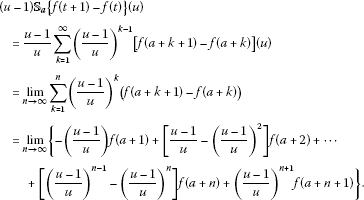Thus, we have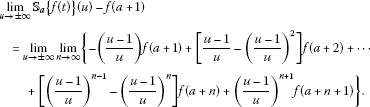Since the series converges uniformly, we can interchange the order of the limits on the right-hand side of the above equality. Thus, we have

$\underset{u\to ±\mathrm{\infty }}{lim}{\mathbb{S}}_{a}\left\{f\left(t\right)\right\}-f\left(a+1\right)=\underset{n\to \mathrm{\infty }}{lim}-f\left(a+1\right)+f\left(a+n+1\right).$

Hence,

$\underset{u\to ±\mathrm{\infty }}{lim}{\mathbb{S}}_{a}\left\{f\left(t\right)\right\}=\underset{t\to \mathrm{\infty }}{lim}f\left(t\right).$

□

Theorem 4.9 (Uniqueness theorem)

Let $f:{\mathbb{N}}_{a}\to \mathbb{R}$ be a function. Then ${\mathbb{S}}_{a}\left\{f\right\}\left(u\right)\equiv 0$ if and only if $f\left(t\right)=0$, $\mathrm{\forall }t\in {\mathbb{N}}_{a+1}$.

Proof If $f\left(t\right)=0$, $\mathrm{\forall }t\in {\mathbb{N}}_{a+1}$, then clearly, by the definition of the Sumudu transform, ${\mathbb{S}}_{a}\left\{f\right\}\left(u\right)=0$. Suppose that ${\mathbb{S}}_{a}\left\{f\right\}\left(u\right)=0$. Then we have

$\frac{1}{u}\left[f\left(a+1\right)+\left(\frac{u-1}{u}\right)f\left(a+2\right)+\cdots +{\left(\frac{u-1}{u}\right)}^{n}f\left(a+n+1\right)+\cdots \right]=0.$
(4.1)

Since the series converges uniformly, taking the limit of the left side as $u\to 1$, we get $f\left(a+1\right)=0$.

Dividing (4.1) by $u-1$ and taking the limit as $u\to 1$, we get $f\left(a+2\right)=0$. Continuing this, we obtain

$f\left(a+1\right)=f\left(a+2\right)=\cdots =0.$

□

Corollary 4.10 Let $f,g:{\mathbb{N}}_{a}\to \mathbb{R}$ be such that ${\mathbb{S}}_{a}\left\{f\left(t\right)\right\}$ and ${\mathbb{S}}_{a}\left\{g\left(t\right)\right\}$ exist and ${\mathbb{S}}_{a}\left\{f\left(t\right)\right\}={\mathbb{S}}_{a}\left\{g\left(t\right)\right\}$, then $f\left(t\right)=g\left(t\right)$, $\mathrm{\forall }t\in {\mathbb{N}}_{a+1}$.

Proof By the linearity it is obvious. □

Below we state the definition of the Taylor monomials which are very useful for applying the Sumudu transform in discrete fractional calculus.

Definition 4.11 

For each $\alpha \in \mathbb{R}\setminus \left\{-1,-2,-3,\dots \right\}$, define the α th-Taylor monomial to be

The following lemma is crucial for finding the Sumudu transform of fractional order nabla Taylor monomials.

Lemma 4.12 

For $\alpha \in \mathbb{R}\setminus \left\{0,±1,±2,\dots \right\}$ and $n\in {\mathbb{N}}_{0}$, we have that

${\left(1+\alpha \right)}^{\overline{n}}=\frac{{\left(-1\right)}^{n}\mathrm{\Gamma }\left(-\alpha \right)}{\mathrm{\Gamma }\left(-\alpha -n\right)}.$

Theorem 4.13 For $\alpha \in \mathbb{R}\setminus \left\{-1,-2,-3,\dots \right\}$, we have

${\mathbb{S}}_{a}\left\{{h}_{\alpha }\left(\cdot ,a\right)\right\}\left(u\right)={u}^{\alpha }\phantom{\rule{1em}{0ex}}\mathit{\text{for}}|\frac{u-1}{u}|<1.$

Proof By Lemma 4.12, we have for $\alpha \in \mathbb{R}\setminus \left\{0,±1,±2,\dots \right\}$

where the last equality follows from the generalized binomial theorem.

Now, when $\alpha =n\in {\mathbb{N}}_{0}$, the proof follows by induction on n. For $n=0$, we have

${\mathbb{S}}_{a}\left\{{h}_{0}\left(\cdot ,a\right)\right\}\left(u\right)={\mathbb{S}}_{a}\left\{1\right\}\left(u\right)=\frac{1}{u}\sum _{k=1}^{\mathrm{\infty }}{\left(\frac{u-1}{u}\right)}^{k-1}=1.$

Suppose for $0\le n\le m$ that ${\mathbb{S}}_{a}\left\{{h}_{n}\left(\cdot ,a\right)\right\}\left(u\right)={u}^{n}$. Then by using the integration by parts formula for nabla time scale, we have

$\begin{array}{rcl}{\mathbb{S}}_{a}\left\{{h}_{m+1}\left(\cdot ,a\right)\right\}\left(u\right)& =& \frac{1}{u}\sum _{k=1}^{\mathrm{\infty }}{\left(\frac{u-1}{u}\right)}^{k-1}{h}_{m+1}\left(k+a,a\right)\\ =& \frac{1}{u}\left[-u{\left(\frac{u-1}{u}\right)}^{k}{h}_{m+1}\left(k+a,a\right){|}_{k=0}^{\mathrm{\infty }}\\ +u\sum _{k=1}^{\mathrm{\infty }}{\left(\frac{u-1}{u}\right)}^{k-1}{h}_{m}\left(k+a,a\right)\right].\end{array}$

Because $|\frac{u-1}{u}|<1$, one has ${lim}_{k\to \mathrm{\infty }}{\left(\frac{u-1}{u}\right)}^{k}{h}_{m+1}\left(k+a,a\right)=0$. Moreover, it can be easily observed that ${h}_{m+1}\left(a,a\right)=0$ for $m\in {\mathbb{N}}_{0}$. Hence,

${\mathbb{S}}_{a}\left\{{h}_{m+1}\left(\cdot ,a\right)\right\}\left(u\right)=\sum _{k=1}^{\mathrm{\infty }}{\left(\frac{u-1}{u}\right)}^{k-1}{h}_{m}\left(k+a,a\right)=u{\mathbb{S}}_{a}\left\{{h}_{m}\left(\cdot ,a\right)\right\}\left(u\right)={u}^{m+1}.$

The proof is now complete. □

Definition 4.14 

Define the convolution of two functions $f,g:{\mathbb{N}}_{a}\to \mathbb{R}$ by

(4.2)

Theorem 4.15 

Let $\alpha \in \mathbb{R}\setminus \left\{0,-1,-2,\dots \right\}$ and $f:{\mathbb{N}}_{a}\to \mathbb{R},$ then

${\mathrm{\nabla }}_{a}^{-\alpha }f\left(t\right)=\left({h}_{\alpha -1}\left(\cdot ,a\right)\ast f\left(\cdot \right)\right)\left(t\right).$
(4.3)

Theorem 4.16 Let $f,g:{\mathbb{N}}_{a}\to \mathbb{R}$ be of exponential order $r>0$. Then for all $u\in \mathbb{C}\setminus \left\{0,1\right\}$ such that $|\frac{u-1}{u}|,

${\mathbb{S}}_{a}\left\{f\ast g\right\}\left(u\right)=u{\mathbb{S}}_{a}\left\{f\right\}\left(u\right){\mathbb{S}}_{a}\left\{g\right\}\left(u\right).$
(4.4)

Proof Let f, g, and r be as in the statement of the lemma. Then

$\begin{array}{rcl}{\mathbb{S}}_{a}\left\{f\ast g\right\}\left(u\right)& =& \frac{1}{u}\sum _{k=1}^{\mathrm{\infty }}{\left(\frac{u-1}{u}\right)}^{k-1}\left(f\ast g\right)\left(a+k\right)\\ =& \frac{1}{u}\sum _{k=1}^{\mathrm{\infty }}{\left(\frac{u-1}{u}\right)}^{k-1}\sum _{s=a+1}^{a+k}f\left(a+k-\rho \left(s\right)+a\right)g\left(s\right)\\ =& \frac{1}{u}\sum _{k=1}^{\mathrm{\infty }}\sum _{s=1}^{k}{\left(\frac{u-1}{u}\right)}^{k-1}f\left(k-\rho \left(s\right)+a\right)g\left(a+s\right)\\ =& \frac{1}{u}\sum _{s=1}^{\mathrm{\infty }}\sum _{k=s}^{\mathrm{\infty }}{\left(\frac{u-1}{u}\right)}^{k-1}f\left(k-\rho \left(s\right)+a\right)g\left(a+s\right)\\ =& \frac{1}{u}\sum _{s=1}^{\mathrm{\infty }}{\left(\frac{u-1}{u}\right)}^{s-1}g\left(a+s\right)\sum _{k=1}^{\mathrm{\infty }}{\left(\frac{u-1}{u}\right)}^{k-1}f\left(a+k\right)\\ =& u{\mathbb{S}}_{a}\left\{g\right\}\left(u\right){\mathbb{S}}_{a}\left\{f\right\}\left(u\right)\end{array}$

for all $u\in \mathbb{C}\setminus \left\{0,1\right\}$ such that $|\frac{u-1}{u}|. □

Theorem 4.17 Suppose $f:{\mathbb{N}}_{a}\to \mathbb{R}$ is of exponential order $0 and let $\alpha >0$. Then for all $u\in \mathbb{C}\setminus \left\{0,1\right\}$ such that $|\frac{u-1}{u}|, we have

${\mathbb{S}}_{a}\left\{{\mathrm{\nabla }}_{a}^{-\alpha }f\right\}\left(u\right)={u}^{\alpha }{\mathbb{S}}_{a}\left\{f\right\}\left(u\right).$
(4.5)

Proof

$\begin{array}{rcl}{\mathbb{S}}_{a}\left\{{\mathrm{\nabla }}_{a}^{-\alpha }f\right\}\left(u\right)& =& {\mathbb{S}}_{a}\left\{{h}_{\alpha -1}\left(\cdot ,a\right)\ast f\left(\cdot \right)\right\}\left(u\right)\\ =& u{\mathbb{S}}_{a}\left\{{h}_{\alpha -1}\left(\cdot ,a\right)\right\}\left(u\right){\mathbb{S}}_{a}\left\{f\right\}\left(u\right)\\ =& u{u}^{\alpha -1}{\mathbb{S}}_{a}\left\{f\right\}\left(u\right)\\ =& {u}^{\alpha }{\mathbb{S}}_{a}\left\{f\right\}\left(u\right).\end{array}$

□

Theorem 4.18 For $f:{\mathbb{N}}_{a}\to \mathbb{R}$, we have

${\mathbb{S}}_{a+1}\left\{\mathrm{\nabla }f\right\}\left(u\right)=\frac{1}{u}\left({\mathbb{S}}_{a+1}\left\{f\right\}\left(u\right)-f\left(a+1\right)\right)$

for all $u\in \mathbb{C}\setminus \left\{0,1\right\}$ satisfying

$\underset{k\to \mathrm{\infty }}{lim}{e}_{\ominus \frac{1}{u}}\left(k,0\right)f\left(a+1+k\right)=0.$
(4.6)

Proof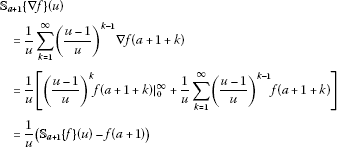provided (4.6) holds. □

We can now generalize this result for an arbitrary positive integer n as follows.

Theorem 4.19 For $f:{\mathbb{N}}_{a}\to \mathbb{R}$, we have

${\mathbb{S}}_{a+N}\left\{{\mathrm{\nabla }}^{N}f\right\}\left(u\right)=\frac{1}{{u}^{N}}{\mathbb{S}}_{a+N}\left\{f\right\}\left(u\right)-\sum _{k=1}^{N}{u}^{k-N-1}{\mathrm{\nabla }}^{k-1}f\left(a+N\right)$

for all $u\in \mathbb{C}\setminus \left\{0,1\right\}$ satisfying

$\underset{k\to \mathrm{\infty }}{lim}{e}_{\ominus \frac{1}{u}}\left(k,0\right){\mathrm{\nabla }}^{i}f\left(a+N+k\right)=0,\phantom{\rule{1em}{0ex}}0\le i\le N-1.$

Proof The proof follows from Theorem 4.18 by applying induction. □

Theorem 4.20 For $f:{\mathbb{N}}_{a}\to \mathbb{R}$, we have

${\mathbb{S}}_{a+N}\left\{f\right\}\left(u\right)={\left(\frac{u}{u-1}\right)}^{N}{\mathbb{S}}_{a}\left\{f\right\}\left(u\right)-\frac{1}{u}\sum _{k=0}^{N-1}{\left(\frac{u}{u-1}\right)}^{N-k}f\left(a+1+k\right).$

Proof

$\begin{array}{rcl}{\mathbb{S}}_{a+N}\left\{f\right\}\left(u\right)& =& \frac{1}{u}\sum _{k=1}^{\mathrm{\infty }}{\left(\frac{u-1}{u}\right)}^{k-1}f\left(a+n+k\right)=\frac{1}{u}\sum _{k=N+1}^{\mathrm{\infty }}{\left(\frac{u-1}{u}\right)}^{k-N-1}f\left(a+k\right)\\ =& \frac{1}{u}\left[\sum _{k=1}^{\mathrm{\infty }}{\left(\frac{u-1}{u}\right)}^{k-N-1}f\left(a+k\right)-\sum _{k=1}^{N}{\left(\frac{u-1}{u}\right)}^{k-N-1}f\left(a+k\right)\right]\\ =& {\left(\frac{u}{u-1}\right)}^{n}{\mathbb{S}}_{a}\left\{f\right\}\left(u\right)-\sum _{k=1}^{n}\frac{{u}^{N-k}}{{\left(u-1\right)}^{N-k+1}}f\left(a+k\right)\\ =& {\left(\frac{u}{u-1}\right)}^{n}{\mathbb{S}}_{a}\left\{f\right\}\left(u\right)-\frac{1}{u}\sum _{k=0}^{N-1}{\left(\frac{u}{u-1}\right)}^{N-k}f\left(a+1+k\right).\end{array}$

□

The following theorem presents the Sumudu transform of the nabla fractional difference of a function.

Theorem 4.21 For $f:{\mathbb{N}}_{a}\to \mathbb{R}$, we have

$\begin{array}{rcl}{\mathbb{S}}_{a+N}\left\{{\mathrm{\nabla }}_{a}^{\alpha }f\right\}\left(u\right)& =& \frac{1}{{u}^{\alpha }}{\left(\frac{u}{u-1}\right)}^{N}{\mathbb{S}}_{a}\left\{f\right\}\left(u\right)-\frac{1}{{u}^{N+1}}\sum _{k=0}^{N-1}{\left(\frac{u}{u-1}\right)}^{N-k}{\mathrm{\nabla }}_{a}^{-\left(N-\alpha \right)}f\left(a+k+1\right)\\ -\sum _{k=0}^{n-1}{u}^{-k-1}\left({\mathrm{\nabla }}^{N-k-1}{\mathrm{\nabla }}_{a}^{-\left(N-\alpha \right)}f\right)\left(a+N\right),\end{array}$

for all $u\in \mathbb{C}\setminus \left\{0,1\right\}$ satisfying

$\underset{k\to \mathrm{\infty }}{lim}{e}_{\ominus \frac{1}{u}}\left(k,0\right)\left({\mathrm{\nabla }}^{i}{\mathrm{\nabla }}_{a}^{-\left(N-\alpha \right)}f\right)\left(a+n+k\right)=0,\phantom{\rule{1em}{0ex}}0\le i\le n-1.$

Proof The proof can be done by replacing f by ${\mathrm{\nabla }}_{a}^{-\left(N-\alpha \right)}f$ in Theorem 4.19 and then using Theorem 4.17. □

In the following theorem, the Sumudu transform of the Caputo nabla fractional difference is given.

Theorem 4.22 For $f:{\mathbb{N}}_{a}\to \mathbb{R}$, we have

$\begin{array}{rcl}{\mathbb{S}}_{a+N}{\left\{}^{C}{\mathrm{\nabla }}_{a}^{\alpha }f\right\}\left(u\right)& =& \frac{1}{{u}^{\alpha }}\left[{\left(\frac{u}{u-1}\right)}^{N}{\mathbb{S}}_{a}\left\{f\right\}\left(u\right)-\frac{1}{u}\sum _{k=0}^{N-1}{\left(\frac{u}{u-1}\right)}^{N-k}f\left(a+1+k\right)\\ -\sum _{k=1}^{N}{u}^{k-N-1}{\mathrm{\nabla }}^{k-1}f\left(a+N\right)\right]\end{array}$

for all $u\in \mathbb{C}\setminus \left\{0,1\right\}$ satisfying

$\underset{k\to \mathrm{\infty }}{lim}{e}_{\ominus \frac{1}{u}}\left(k,0\right){\mathrm{\nabla }}^{i}f\left(a+N+k\right)=0,\phantom{\rule{1em}{0ex}}0\le i\le N-1.$

Proof

$\begin{array}{rcl}{\mathbb{S}}_{a+N}{\left\{}^{C}{\mathrm{\nabla }}_{a}^{\alpha }f\right\}\left(u\right)& =& {\mathbb{S}}_{a+N}\left\{{\mathrm{\nabla }}_{a+N}^{-\left(N-\alpha \right)}{\mathrm{\nabla }}^{N}f\right\}\left(u\right)\\ =& \frac{1}{u}\sum _{k=1}^{\mathrm{\infty }}{\left(\frac{u-1}{u}\right)}^{k-1}{\mathrm{\nabla }}_{a+N}^{-\left(N-\alpha \right)}{\mathrm{\nabla }}^{N}f\left(a+N+k\right)\\ =& \frac{1}{u}\sum _{k=1}^{\mathrm{\infty }}{\left(\frac{u-1}{u}\right)}^{k-1}\sum _{s=a+N+1}^{a+N+k}\frac{{\left(a+N+k-\rho \left(s\right)\right)}^{\overline{N-\alpha -1}}}{\mathrm{\Gamma }\left(N-\alpha \right)}{\mathrm{\nabla }}^{N}f\left(s\right)\\ =& \frac{1}{u}\sum _{k=1}^{\mathrm{\infty }}\sum _{s=1}^{k}{\left(\frac{u-1}{u}\right)}^{k-1}\frac{{\left(k-\rho \left(s\right)\right)}^{\overline{N-\alpha -1}}}{\mathrm{\Gamma }\left(N-\alpha \right)}{\mathrm{\nabla }}^{N}f\left(a+N+s\right)\\ =& \frac{1}{u}\sum _{s=1}^{\mathrm{\infty }}\sum _{k=s}^{\mathrm{\infty }}{\left(\frac{u-1}{u}\right)}^{k-1}\frac{{\left(k-\rho \left(s\right)\right)}^{\overline{N-\alpha -1}}}{\mathrm{\Gamma }\left(N-\alpha \right)}{\mathrm{\nabla }}^{N}f\left(a+N+s\right)\\ =& \frac{1}{u}\sum _{s=1}^{\mathrm{\infty }}\sum _{k=1}^{\mathrm{\infty }}{\left(\frac{u-1}{u}\right)}^{k+s-2}\frac{{k}^{\overline{N-\alpha -1}}}{\mathrm{\Gamma }\left(N-\alpha \right)}{\mathrm{\nabla }}^{N}f\left(a+N+s\right)\\ =& \frac{1}{u}\sum _{k=1}^{\mathrm{\infty }}{\left(\frac{u-1}{u}\right)}^{k-1}\frac{{k}^{\overline{N-\alpha -1}}}{\mathrm{\Gamma }\left(N-\alpha \right)}\sum _{s=1}^{\mathrm{\infty }}{\left(\frac{u-1}{u}\right)}^{s-1}{\mathrm{\nabla }}^{N}f\left(a+N+s\right)\\ =& u{\mathbb{S}}_{a}\left\{{h}_{N-\alpha -1}\left(\cdot ,a\right)\right\}\left(u\right){\mathbb{S}}_{a+N}\left\{{\mathrm{\nabla }}^{N}f\right\}\left(u\right)\\ =& {u}^{N-\alpha }{\mathbb{S}}_{a+N}\left\{{\mathrm{\nabla }}^{N}f\right\}\left(u\right)\\ =& {u}^{N-\alpha }\left[\frac{1}{{u}^{N}}{\mathbb{S}}_{a+N}\left\{f\right\}\left(u\right)-\sum _{k=1}^{N}{u}^{k-N-1}{\mathrm{\nabla }}^{k-1}f\left(a+N\right)\right]\\ =& \frac{1}{{u}^{\alpha }}\left[{\left(\frac{u}{u-1}\right)}^{N}{\mathbb{S}}_{a}\left\{f\right\}\left(u\right)-\frac{1}{u}\sum _{k=0}^{N-1}{\left(\frac{u}{u-1}\right)}^{N-k}f\left(a+1+k\right)\\ -\sum _{k=1}^{N}{u}^{k-N-1}{\mathrm{\nabla }}^{k-1}f\left(a+N\right)\right].\end{array}$

□

The discrete Mittag-Leffler function was defined in  by

${E}_{p,\alpha ,\beta }\left(t,a\right)=\sum _{k=0}^{\mathrm{\infty }}{p}^{k}{h}_{\alpha k+\beta }\left(t,a\right),$
(4.7)

where $\alpha >0$, $\beta \in \mathbb{R}$ and $|p|<1$.

In the following theorem, we establish the Sumudu transform of the Mittag-Leffler function.

Theorem 4.23 For $|P|<1$, $\alpha >0$, $\beta \in \mathbb{R}$ and $|p{u}^{\alpha }|<1$, we have

${\mathbb{S}}_{a}\left\{{E}_{p,\alpha ,\beta }\left(t,a\right)\right\}\left(u\right)=\frac{{u}^{\beta }}{1-p{u}^{\alpha }}.$

Proof

${\mathbb{S}}_{a}\left\{{E}_{p,\alpha ,\beta }\left(t,a\right)\right\}\left(u\right)=\sum _{k=0}^{\mathrm{\infty }}{p}^{k}{S}_{a}\left\{{h}_{\alpha k+\beta }\left(t,a\right)\right\}=\sum _{k=0}^{\mathrm{\infty }}{p}^{k}{u}^{\alpha k+\beta }=\frac{{u}^{\beta }}{1-p{u}^{\alpha }}.$

□

## 5 Application

In this section, we will illustrate the possible use of the discrete Sumudu transform by applying it to solve some initial value problems.

Example 5.1

Consider the initial value problem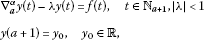where $y,f:{\mathbb{N}}_{a}\to \mathbb{R}$ and $0<\alpha <1$.

We begin by taking the Sumudu transform of both sides of the equation, starting at $a+1$. Keeping in mind that ${\mathrm{\nabla }}_{a}^{-\left(1-\alpha \right)}y\left(t\right)=y\left(a+1\right)={y}_{0}$, by using Theorem 4.20 and Theorem 4.21, we get

$\begin{array}{rcl}{\mathbb{S}}_{a}\left\{y\right\}\left(u\right)& =& \left[\left(1-\lambda \right){y}_{0}-f\left(a+1\right)\right]\frac{{u}^{\alpha -1}}{1-\lambda {u}^{\alpha }}+u{\mathbb{S}}_{a}f\left(u\right)\frac{{u}^{\alpha -1}}{1-\lambda {u}^{\alpha }}\\ =& \left[\left(1-\lambda \right){y}_{0}-f\left(a+1\right)\right]{\mathbb{S}}_{a}\left\{{E}_{\lambda ,\alpha ,\alpha -1}\left(t,a\right)\right\}\left(u\right)+u{\mathbb{S}}_{a}\left\{f\right\}\left(u\right){\mathbb{S}}_{a}\left\{{E}_{\lambda ,\alpha ,\alpha -1}\left(t,a\right)\right\}\left(u\right)\\ =& \left[\left(1-\lambda \right){y}_{0}-f\left(a+1\right)\right]{\mathbb{S}}_{a}\left\{{E}_{\lambda ,\alpha ,\alpha -1}\left(t,a\right)\right\}\left(u\right)+{\mathbb{S}}_{a}\left\{f\left(t\right)\ast {E}_{\lambda ,\alpha ,\alpha -1}\left(t,a\right)\right\}\left(u\right).\end{array}$

Thus, we have

$y\left(t\right)=\left[\left(1-\lambda \right){y}_{0}-f\left(a+1\right)\right]{E}_{\lambda ,\alpha ,\alpha -1}\left(t,a\right)+f\left(t\right)\ast {E}_{\lambda ,\alpha ,\alpha -1}\left(t,a\right).$

Example 5.2

Consider the initial value problem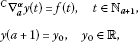where $y,f:{\mathbb{N}}_{a}\to \mathbb{R}$ and $0<\alpha \le 1$.

Taking the Sumudu transform of both sides of the equation, starting at $a+1$, and using Theorem 4.20 and Theorem 4.22, we get

$\begin{array}{rcl}{\mathbb{S}}_{a}\left\{y\right\}\left(u\right)& =& {y}_{0}+{u}^{\alpha }{\mathbb{S}}_{a}\left\{f\right\}\left(u\right)-{u}^{\alpha -1}f\left(a+1\right)\\ =& {y}_{0}{\mathbb{S}}_{a}\left\{1\right\}\left(u\right)+u{\mathbb{S}}_{a}\left\{{h}_{\alpha -1}\left(t,a\right)\right\}\left(u\right){\mathbb{S}}_{a}\left\{f\right\}\left(u\right)-f\left(a+1\right){\mathbb{S}}_{a}\left\{{h}_{\alpha -1}\left(t,a\right)\right\}\left(u\right).\end{array}$

Therefore, we have

$\begin{array}{rcl}y\left(t\right)& =& {y}_{0}+{h}_{\alpha -1}\left(t,a\right)\ast f\left(t\right)-f\left(a+1\right){h}_{\alpha -1}\left(t,a\right)\\ =& {y}_{0}+\sum _{s=a+1}^{t}\frac{{\left(t-\rho \left(s\right)\right)}^{\overline{\alpha -1}}}{\mathrm{\Gamma }\left(\alpha \right)}f\left(s\right)-f\left(a+1\right)\frac{{\left(t-\rho \left(s\right)\right)}^{\overline{\alpha -1}}}{\mathrm{\Gamma }\left(\alpha \right)}\\ =& {y}_{0}+\sum _{s=a+2}^{t}\frac{{\left(t-\rho \left(s\right)\right)}^{\overline{\alpha -1}}}{\mathrm{\Gamma }\left(\alpha \right)}f\left(s\right)\\ =& {y}_{0}+{\mathrm{\nabla }}_{a+1}^{-\alpha }f\left(t\right).\end{array}$

## References

1. Heymans N, Podlubny I: Physical interpretation of initial conditions for fractional differential equations with Riemann-Liouville fractional derivatives. Rheol. Acta 2006, 45: 765–771. 10.1007/s00397-005-0043-5

2. Kilbas AA, Srivastava HH, Trujillo JJ: Theory and Applications of Fractional Differential Equations. Elsevier, Amsterdam; 2006.

3. Magin RL: Fractional Calculus in Bioengineering. Begell House Publisher, Connecticut; 2006.

4. Podlubny I: Fractional Differential Equations. Academic Press, San Diego; 1999.

5. Samko SG, Kilbas AA, Marichev OI: Fractional Integrals and Derivatives - Theory and Applications. Gordon & Breach, Linghorne; 1993.

6. West BJ, Bologna M, Grigolini P: Physics of Fractal Operators. Springer, New York; 2003.

7. Miller KS, Ross B: Fractional difference calculus. Proceedings of the International Symposium on Univalent Functions, Fractional Calculus and Their Applications 1989, 139–152.

8. Abdeljawad T, Baleanu D: Fractional differences and integration by parts. J. Comput. Anal. Appl. 2011, 13(3):574–582.

9. Abdeljawad T: On Riemann and Caputo fractional differences. Comput. Math. Appl. 2011, 62(3):1602–1611. 10.1016/j.camwa.2011.03.036

10. Abdeljawad, T: Principles of delta and nabla fractional differences. arXiv:1112.5795v1 (2011)

11. Atici FM, Eloe PW: A transform method in discrete fractional calculus. Int. J. Differ. Equ. Appl. 2007, 2(2):165–176.

12. Atici FM, Eloe PW: Initial value problems in discrete fractional calculus. Proc. Am. Math. Soc. 2009, 137(3):981–989.

13. Atici FM, Eloe PW: Discrete fractional calculus with the nabla operator. Electron. J. Qual. Theory Differ. Equ. 2009, 2009(3):1–12.

14. Ahrendt, K, Castle, L, Holm, M, Yochman, K: Laplace transforms for the nabla-difference operator and a fractional variation of parameters formula. Commun. Appl. Anal. (to appear)

15. Holm, MT: The theory of discrete fractional calculus: development and application. PhD thesis, University of Nebraska (2011)

16. Hein J, McCarthy Z, Gaswick N, McKain B, Speer K: Laplace transforms for the nabla-difference operator. Panam. Math. J. 2011, 21(3):79–97.

17. Watugala GK: Sumudu transform: a new integral transform to solve differential equations and control engineering problems. Int. J. Math. Educ. Sci. Technol. 1993, 24(1):35–43. 10.1080/0020739930240105

18. Watugala GK: The Sumudu transform of functions of two variables. Math. Eng. Ind. 1998, 8(4):293–302.

19. Asiru MA: Sumudu transform and the solution of integral equations of convolution type. Int. J. Math. Educ. Sci. Technol. 2001, 32(6):906–910. 10.1080/002073901317147870

20. Asiru MA: Further properties of the Sumudu transform and its applications. Int. J. Math. Educ. Sci. Technol. 2001, 33(2):441–449.

21. Belgacem FBM, Karballi AA, Kalla LS: Analytic investigations of the Sumudu transform and applications to integral production equations. Math. Probl. Eng. 2003, 3: 103–118.

22. Belgacem FBM, Karballi AA: Sumudu transform fundamental properties investigations and applications. J. Appl. Math. Stoch. Anal. 2006, 2006: 1–23.

23. Kılıçman A, Eltayeb H: On the applications of Laplace and Sumudu transforms. J. Franklin Inst. 2010, 347(5):848–862. 10.1016/j.jfranklin.2010.03.008

24. Belgacem FBM: Introducing and analysing deeper Sumudu properties. Nonlinear Stud. 2006, 13(1):23–41.

25. Jarad F, Tas K: Application of Sumudu and double Sumudu transforms to Caputo-fractional differential equations. J. Comput. Anal. Appl. 2012, 14(3):475–483.

26. Katatbeh QD, Belgacem FBM: Applications of the Sumudu transform to fractional differential equations. Nonlinear Stud. 2011, 18(1):99–112.

27. Jarad F, Tas K: On Sumudu transform method in discrete fractional calculus. Abstr. Appl. Anal. 2012., 2012: Article ID 270106. doi:10.1155/2012/270106

28. Bohner M, Guseinov GS: The h -Laplace and q -Laplace transforms. J. Math. Anal. Appl. 2010, 365: 75–92. 10.1016/j.jmaa.2009.09.061

29. Hilger S: Analysis on measure chains - a unified approach to continuous and discrete calculus. Results Math. 1990, 18: 18–56.

30. Bohner M, Peterson A: Dynamic Equations on Time Scales. Birkhäuser, Boston; 2001.

31. Bohner M, Peterson A: Advances in Dynamic Equations on Time Scales. Birkhäuser, Boston; 2003.

32. Anastassiou G: Nabla discrete fractional calculus and nabla inequalities. Math. Comput. Model. 2010, 51: 562–571. 10.1016/j.mcm.2009.11.006

## Acknowledgements

The authors would like to thank the reviewers for their valuable comments.

## Author information

Authors

### Corresponding author

Correspondence to Billur Kaymakçalan.

### Competing interests

The authors declare that they have no competing interests.

### Authors’ contributions

All authors contributed equally and significantly in writing this paper.

## Rights and permissions

Reprints and Permissions

Jarad, F., Kaymakçalan, B. & Taş, K. A new transform method in nabla discrete fractional calculus. Adv Differ Equ 2012, 190 (2012). https://doi.org/10.1186/1687-1847-2012-190

• Accepted:

• Published:

• DOI: https://doi.org/10.1186/1687-1847-2012-190

### Keywords

• discrete Sumudu transform
• fractional sums
• fractional differences
• convolution
• time scale